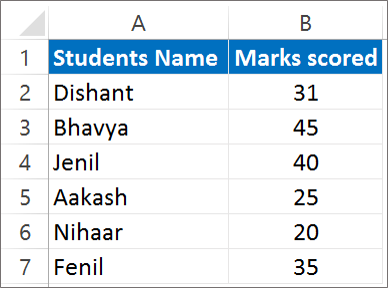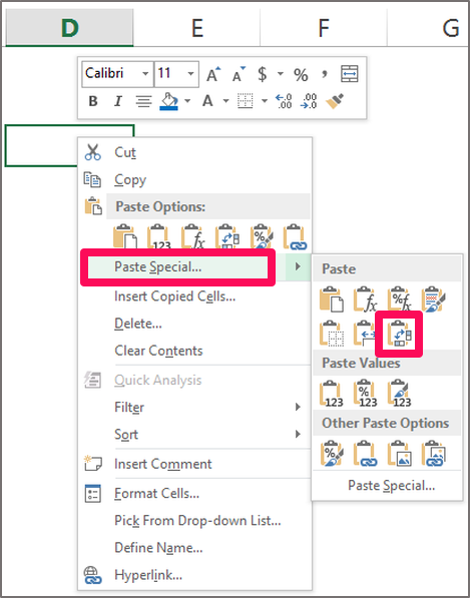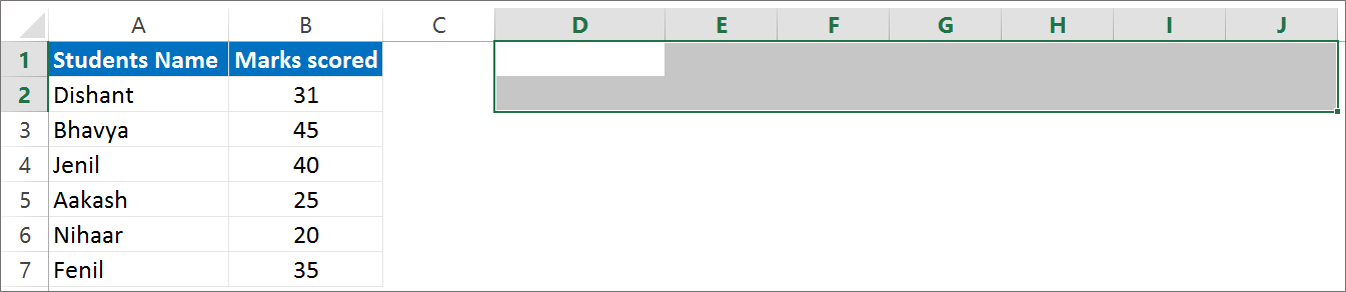Need Help? Chat with us

Excel Transpose Formula | switch excel Columns to Rows

Excel Transpose Formula | How to switch rows in excel

This function gives an advantage of turning the way the data is presented in the worksheet. Excel transpose Formula converts a horizontal data into vertical one or vertical data into horizontal one. The Transpose Function is one such option for changing the way data is presented.

You can flip your data located in rows to columns or columns to rows. You can transpose a single row or column or multiple set of rows or column using the Transpose function.

The main purpose of this function is to flip the range of cells. It returns in a new oriented format. This function is also at in-built function in Excel categorized under Lookup/Reference Function. It is a worksheet function in Excel so it can be entered as a part of the formula. This function applied to all the Excel version from 2000 to 2016.

There are two ways you can use Transpose function in Excel. The first way is to use the Copy and Paste Option to transpose data and the second way is to use the Excel Transpose formula. This tutorial will explain how you can transpose data with this two ways.

Transpose Data using Copy and Paste Special option

One of the way to use transpose data is to use copy and paste special option. The steps to Transpose data using Copy and paste special is as follow:

Step 1: Select the range of cells that you want to transpose. Here A1: B7 is selected.Step 2: Right click on the Mouse and Select Copy. and Select the cell where you want to Paste the copied Data. Here D3 is selected. Right Click on the Mouse and Go to Paste Special > Select TransposeResult: You will see that your data will be transpose. Vertical data will be converted into horizontal data in our case.Note: When you transpose data, both the sets are linked to each other. So, a change in one data will automatically make change in the other set of data. However this trick won’t work when you have used the copy and paste special option to transpose data. It works only while transposing data using the Excel transpose formula.

Transpose Data using Excel Transpose Formula

The Syntax of Transpose function in Excel is:

TRANSPOSE(array)

This function requires only one argument. That is the array argument. In the array argument you enter the range of cells that you want to transpose.

The steps to transpose data using the Transpose formula is as follow:

Step 1: Select the range of cell. The range selected should be equivalent to the range we are going to transpose. In our Example we have 2 columns and 7 rows. To Transpose data we will have to select 2 rows and 7 columns.Step 2: Type the Transpose Formula =TRANSPOSE( A1:B7) and than Press CTRL + SHIFT + ENTER.Note: Make sure you don’t press Enter after you enter the formula. You need to keep in mind that you should press CTRL + SHIFT + ENTER.

How will you know whether the formula entered is an array formula or no? You can see in the formula bar that the formula is covered with a curly bracket. This indicates that this is an array formula.

Note: You cannot enter the curly brackets by yourself while entering the formula. The curly brackets will be entered automatically after you press CTRL + SHIFT + ENTER.

Hope you like our tutorial on Excel Transpose Formula for more cool and amazing trick of excel Like our Excel Superstar Facebook Page and subscribe to our Excel Superstar YouTube channel. Excel Superstar is the leading online training company, which provides Online Excel Course in Hindi. Connect with us and become an Excel Superstar.